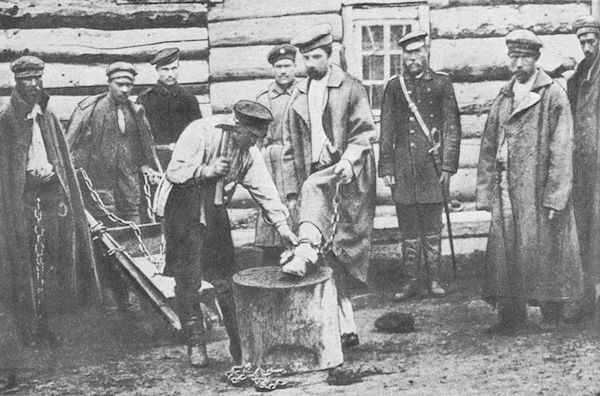# Lies, Lies

Probability Level 5There are three prisoners in a prison. Prisoner 1 tells the truth $20$% of the time and lies the rest of the time, Prisoner 2 tells the truth or a lie with equal probability, and Prisoner 3 tells the truth $\dfrac{9}{10}$ of the time, and lies the rest. You decide to play a game. You arrange the prisoners in a line like so: ${\text{Prisoner 1}, \text{Prisoner 2}, \text{Prisoner 3}}$. You go down the line, starting from Prisoner 1, and ask each of them a question. Whenever a prisoner tells the truth, you let them go, until only one remains. When the probability that Prisoner 1 is let go is expressed as a fraction in the form $\dfrac{a}{b}$ for coprime positive integers $a$ and $b$, what is the value of $a+b$?

×

Problem Loading...

Note Loading...

Set Loading...